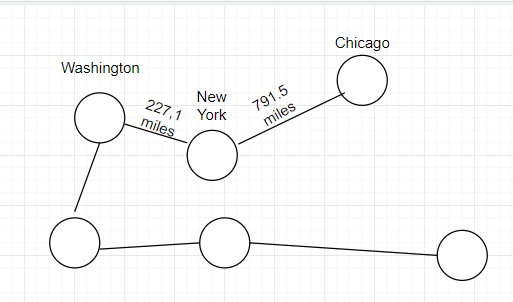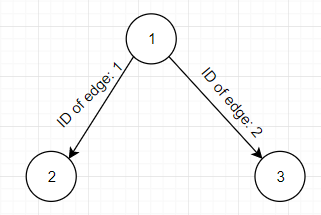# Graphs Java Example

Graphs are usually made from vertices and arcs. Sometimes they are also called nodes (instead of vertices) and edges (instead of arcs). For the sake of this tutorial I will be using nodes and edges as reference.Graphs usually look something like this:

In many cases, the nodes and the edges are assigned values to them. A famous example of a graph that is very useful is, when nodes represent cities and the edges represent distance between these 2 nodes (or cities for that matter). Such an example can be seen below:Judging by the image above, it is very easy to understand what it represents and is very easy to read. The distance between Chicago and New York is 791.5 miles and the distance between New York and Washington DC is 227.1 miles.

This is just 1 simple example of how using a graph could be useful, but there are many more.

Other examples of graph being useful could be representing family tree, facebook contacts, even travel routes.

## Undirected Graph

When a graph is undirected, that means that the edges can be traversed in both directions.

## Directed Graph

When a graph is directed, that means that the edges can be traversed only in the direction they are “pointing to”.

## Graph implementation in Java

Node.java

```import java.util.*;

public class Node {
private int id;
private List<Edge> neighbours = new ArrayList<Edge>();

public int getNodeId() {
return this.id;
}

if(this.neighbours.contains(e)) {
System.out.println("This edge has already been used for this node.");
} else {
}
}

public void getNeighbours() {
System.out.println("List of all edges that node " + this.id +" has: ");
System.out.println("=================================");
for (int i = 0; i < this.neighbours.size(); i++ ){
System.out.println("ID of Edge: " + neighbours.get(i).getId() + "\nID of the first node: " + neighbours.get(i).getIdOfStartNode() +
"\nID of the second node: " + neighbours.get(i).getIdOfEndNode());
System.out.println();
}
System.out.println(neighbours);
}

public Node(int id) {
this.id = id;
}
}```

Node.java has 3 methods and 1 constructor.

getNodeId() simply returns each node’s id.

addNeighbour(Edge e) creates a connection via an edge which is passed as a parameter to another node. It is done by adding the specified edge to the List of edges in the Node class. Note that there is an if condition that checks if the specified edge e already exists in the current edges of this node.

getNeighbours() is used just for displaying purposes. View the output to see how exactly this method displays the information.

The constructor takes id as a parameter.

Edge.java

```public class Edge {
private Node start;
private Node end;
private double weight;
private int id;

public int getId() {
return this.id;
}

public Node getStart() {
return this.start;
}

public int getIdOfStartNode() {
return this.start.getNodeId();
}

public Node getEnd() {
return this.end;
}

public int getIdOfEndNode() {
return this.end.getNodeId();
}

public double getWeight() {
return this.weight;
}

public Edge(Node s, Node e, double w, int id) {
this.start = s;
this.end = e;
this.weight = w;
this.id = id;
}
}
```

Edge.java has 6 methods and 1 constructor.

getId() simply returns the id of the current edge.

getStart() returns the Node object from which the edge starts.

getIdOfStartNode() returns the id of the Node object from which the edge starts.

getEnd() returns the Node object that the edge “stops” at.

getIdOfEndNode() returns the id of the Node object that the edge “stops” at.

getWeight() gets the weight of the current Node object.

The Edge constructor takes 4 parameters and initializes the constructor using them.

Graph.java

```import java.util.*;

public class Graph {
private List<Node> nodes = new ArrayList<Node>();
private int numberOfNodes = 0;

public boolean checkForAvailability() { // will be used in Main.java
return this.numberOfNodes > 1;
}

public void createNode(Node node) {
this.numberOfNodes++; // a node has been added
}

public int getNumberOfNodes() {
return this.numberOfNodes;
}
}```

Graph.java has only 3 methods and no constructor.

checkForAvailability() checks if there are more than 1 node. If there aren’t any more than 1 node, then a connection cannot be made as a node cannot have an edge towards itself. It has to have a connection with another node.

createNode(Node node) takes an argument of type Node and adds that node to the nodes List. After the node has been added, the current graph increments the number of nodes by 1. That way, we can evaluate the checkForAvailability() method to true at some point.

getNumberOfNodes() returns the number of nodes.

Main.java

```public class Main {
public static void main(String args[]) {
Graph graph = new Graph();

Node node1 = new Node(1); // create a new node that contains id of 1
Node node2 = new Node(2); // create a new node that contains id of 2
Node node3 = new Node(3); // create a new node that contains id of 3

graph.createNode(node1); // numberOfNodes should increment by 1
graph.createNode(node2); // numberOfNodes should increment by 1
graph.createNode(node3); // numberOfNodes should increment by 1

Edge e12 = new Edge(node1, node2, 5, 1); // create an edge that connects node1 to node2 and contains weight of 5
Edge e13 = new Edge(node1, node3, 10, 2); // create an edge that connects node1 to node3 and contains weight of 10

if (graph.checkForAvailability()) {
// two nodes can be connected via edge
node1.addNeighbour(e12); // connect 1 and 2 (nodes)
node1.getNeighbours();
} else {
System.out.println("There are less than 2 nodes. Add more to connect.");
}
}
}
```

Main.java has only a main method.

A graph is created within the main method. After which, 3 instances of Node are created. Then, these Node instances are added to the graph using the createNode(Node node) method. After that, 2 instances of Edge are created. The first, connects node1 to node 2. The second, connects node1 to node3.

After that, there is an if condition that checks if the number of nodes is more than 1 and if it is, add the “Neighbour” to node1. (e12 is the edge that connects node1 and node2.) (e13 is the edge that connects node1 and node3).

Output

```Successfully added Edge@15db9742

List of all edges that node 1 has:
=================================
ID of Edge: 1
ID of the first node: 1
ID of the second node: 2

ID of Edge: 2
ID of the first node: 1
ID of the second node: 3

[Edge@15db9742, Edge@6d06d69c]```

Visualization of the above output:Question: is the above program producing an undirected or directed graph? If it produces unidrected graph, can you modify the API to produce directed one? And if produces directed graph, can you modify the API to produce undirected one?

Answer: the Graph above produces a directed graph, because as the name suggests, the arcs are “pointing” to a location. To make it a undirected you would simply need to remove the “arrow” of the arcs and just make them as a simple line. Just like the image below that represents the undirected graph.